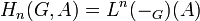# Homology group for trivial group action

## Definition

Let$G$ be a group and$A$ be an abelian group.

The homology groups for trivial group action$\! H_n(G,A)$, also denoted$\! H_n(G;A)$ ($n = 0,1,2,3,\dots$) are abelian groups defined in the following equivalent ways.

### Definition in terms of classifying space$\! H_n(G,A)$ can be defined as the homology group$H_n(BG,A)$, where$BG$ is the classifying space of$G$ and theohomology group is understood to be in the topological sense (singular homology or cellular homology, or any of the equivalent homology theories satisfying the axioms).

### Definition as homology group for an action taken as the trivial action

The homology groups for trivial group action$H_n(G,A)$ are defined as the homology groups$H_{n,\varphi}(G,A)$ where$\varphi:G \to \operatorname{Aut}(A)$ is the trivial map. In other words, we treat$A$ as a$G$-module with trivial action of$G$ on$A$ (i.e., every element of$G$ fixes every element of$A$. We thus also treat$A$ as a trivial$\mathbb{Z}G$-module, where$\mathbb{Z}G$ is a group ring of$G$ over the ring of integers$\mathbb{Z}$.

No. Shorthand Detailed description of$H_{n,\varphi}(G,A)$, the$n^{th}$ homology group
1 Explicit, using the bar resolution$H_n(G,A)$, is defined as the quotient$Z_n(G,A)/B_n(G,A)$ where$Z_n(G,A)$ is the group of cycles for the action and$B_n(G,A)$ is the group of boundaries.
2 Complex based on arbitrary projective resolution Let$\mathcal{F}$ be a projective resolution for$\mathbb{Z}$ as a$\mathbb{Z}G$-module with the trivial action. Let$\mathcal{C}$ be the complex$\mathcal{F} \otimes_{\mathbb{Z}G} A$. The homology group$H_n(G,A)$ is defined as the$n^{th}$ homology group for this complex.
3 As a$\operatorname{Tor}$ functor$\operatorname{Tor}_n^{\mathbb{Z}G}(\mathbb{Z},A)$ where$\mathbb{Z}$ is a trivial$\mathbb{Z}G$-module and$A$ has the module structure specified by$\varphi$.
4 As a left derived functor$H_n(G,A) = L^n(-_G)(A)$, i.e., it is the$n^{th}$ left derived functor of the coinvariants functor for$G$ (denoted$-_G$) evaluated at$A$. The coinvariants functor sends a$\mathbb{Z}G$-module$M$ to$M/N$ where$N$ is generated by all elements of the form$(g-1)m, g\in G, m \in M$.